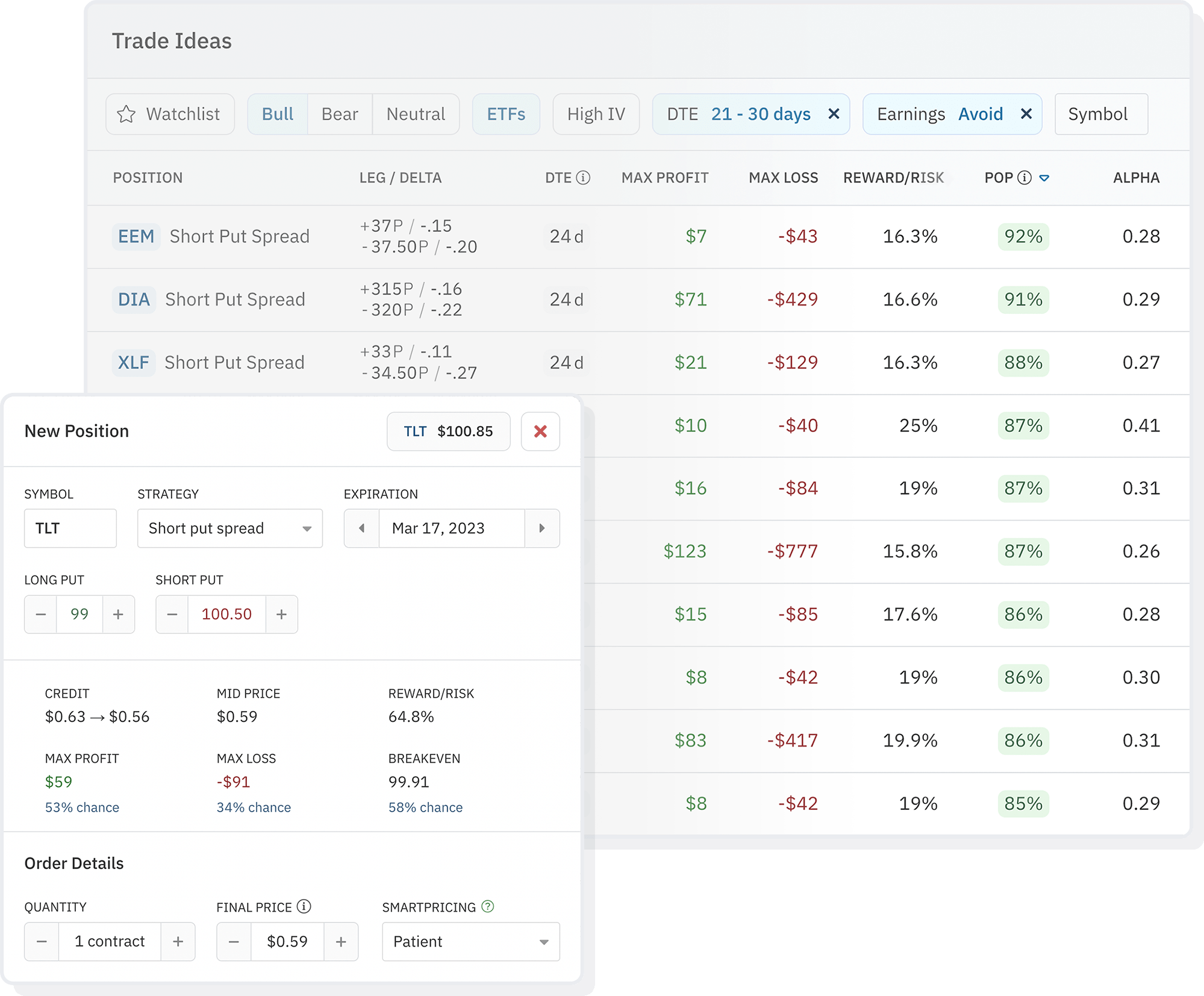Big announcement!

# Backtesting Metrics

Overview of how the autotrading backtest engine operates and what each of the different performance metrics mean.

Option Alpha provides a backtesting tool for idea generation and discovery.

The backtester uses end-of-day (EOD) data, meaning there is only one data point per day per option symbol. Each data point is OPRA (Options Price Reporting Authority) data, captured at approximately 3:45 PM Eastern time every day.

Backtesting data exists back to January 2007. An "All-Time" backtest start date is 1 January 2007. All backtests are pre-run with 1 contract; allocation settings are applied after the fact.

If Avoid Earnings is set, the backtester won't open a position if there is an earnings event during the expiration cycle of the position about to be opened.

## Allocation

The allocation rules are straightforward. Each backtest is given a Starting Capital and at least one (up to three) allocations.

Allocators are rules-based, just like recipes. Unless the criteria are met, a new backtest position won't be opened until capital is freed.

## Parameter Selection

Backtesting parameter selection works in a slightly more restrictive manner than the bots. Each parameter mapping, such as delta, is chosen in the same closest to manner that the bots would use.

However, each parameter type is bound by a unique range. If the next closest value to the chosen parameter value is outside of that range, the backtester will simply not open a position on that day.

#### Date selection

The backtester's selection for the date to open positions will vary depending on the other entry and exit conditions you set.

For example, if when using Sequential frequency, the dates will be one after another and this could vary depending on the duration (DTE) of the contract. If using Weekly the backtester will seek to open a position every seven days, if available.

Ultimately, the various entry and exit conditions, as well as the trade frequency, will all determine the date when positions are opened.

## Stats

• Return on Capital - % return of the initial allocation
• CAGR - Compounded Annual Growth Rate of the initial allocation. Compounded annual growth rate measures the rate an investment would grow if it increased at the same rate yearly and the profits were reinvested at the end of each year.
• Max Drawdown - Largest peak-to-trough change in dollars
• Total P/L - Overall profit or loss in dollars
• Profit Factor - The profit factor divides the total amount of money gained by the total amount lost. The profit factor is important when used with the win rate because it creates a complete view of trading performance.
• Sharpe Ratio - The Sharpe ratio is calculated by subtracting the risk-free rate of return from the portfolio’s return and dividing the value by the portfolio’s standard deviation of returns. The Sharpe ratio helps to determine if returns result from sound investing or a product of taking on too much risk and can be used to evaluate past performance. The higher the Sharpe ratio’s value, the better the performance is relative to the risk required to generate the returns.
• Sortino Ratio -  The Sortino ratio is similar to the Sharpe ratio but focuses primarily on negative volatility in a portfolio. In calculating the standard deviation for the Sortino ratio, only the returns that lie below the mean are used. Therefore, the Sortino ratio allows investors to evaluate the return of investment based on its return for downside risk only.
• Calmer Ratio - The Calmar ratio uses a portfolio’s maximum drawdown as a measure of its risk and compares it to the compounded annual rate of return. The Calmar ratio uses a three-year lookback period and is updated monthly. A high Calmar ratio equates to a low risk of large drawdowns, while a low Calmar ratio indicates a higher risk of a large drawdown.
• Win Rate - The win-rate divides the total number of winning trades by the total trades. For example, if an investor has placed 200 trades and has realized a profit on 120 of them, the win rate is 60% (120/200).
• Expected P/L - Expectancy, the average expected profit per trade. After a backtest has concluded, we can calculate the historical expectancy (as opposed to projected expectancy based on theoretical probability, for example). In other words, given enough occurrences, how much money do we expect to make per trade?

### Expected P/L Per Position

After a backtest has concluded, we can calculate the historical expectancy (as opposed to projected expectancy based on theoretical probability, for example). In other words, given enough occurrences, how much money do we expect to make per trade?

The generic formula is:

## FAQs